# GSEB Solutions Class 10 Maths Chapter 3 Pair of Linear Equations in Two Variables Ex 3.4

Gujarat Board GSEB Solutions Class 10 Maths Chapter 3 Pair of Linear Equations in Two Variables Ex 3.4 Textbook Questions and Answers.

## Gujarat Board Textbook Solutions Class 10 Maths Chapter 3 Pair of Linear Equations in Two Variables Ex 3.4

Question 1.
Solve the following pair of linear equations by the elimination method and the substitution method:

1. x + y = 5 and 2x – 3y = 4
2. 3x + 4y = 10 and 2x – 2y = 2
3. 3x – 5y – 4 = 0 and 9x = 2y + 7
4. $$\frac{x}{2}+\frac{2 y}{3}$$ = -1 and x – $$\frac{y}{3}$$ – 3

Solution:
1. x + y = 5 and 2x – 3y – 4
I. By Elimination Method:
The given system of equation is
x + y = 5 …(1)
2x – 3y = 4 …(2)
Multiplying equation (1) by 3, we get
3x + 3y = 15 …(3)
Adding equation (2) and equation (3), we get
5x = 19
x = $$\frac{19}{5}$$
Substituting this value of x in equation (1), we get
$$\frac{19}{5}$$ + y = 5
⇒ y = 5 – $$\frac{19}{5}$$
⇒ y = $$\frac{6}{5}$$
So, the solution of the given system equations is
$$x=\frac{19}{5}, y=\frac{6}{5}$$II. By Substitution Method:
The given systems of equation is
x + y = 5 …(1)
2x – 3y = 4 …(2)
From equation (1),
y = 5 – x …(3)
Substitute this value ofy in equation (2), we get
2x – 3(5 – x) = 4
⇒ 2x – 15 + 3x = 4
⇒ 5x – 15 = 4
⇒ 5x = 15 + 4
⇒ 5x = 19
⇒ x = $$\frac{19}{5}$$
Substituting this value of* in equation (3),
we getSo, the solution of the given system of equations isVerification:
Substituting x = $$\frac{19}{5}$$ and y = $$\frac{6}{5}$$, we find that both the equations (1) and (2) are satisfied as shown below: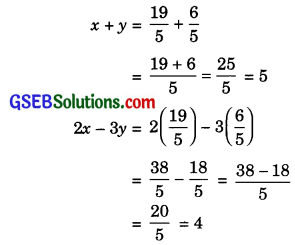Hence, the solution is correct.2. 3x + 4y = 10 and 2x – 2y = 2
I. By Elimination method:
The given system of equation is
3x + 4y = 10 …(1)
2x – 2y = 2 …(2)
Multiplying equation (2), by 2, we get
4x – 4y = 4 …(3)
Adding equation (1) and equation (3), we getSubstituting this value of x in equation (2), we get
2(2) – 2y = 2
⇒ 4 – 2y = 2
⇒ 2y = 4 – 2
⇒ 2y = 2
⇒ y = $$\frac{2}{2}$$ = 1
So, the solution of the given system by equations is x = 2, y = 1.II. By substitution method:
The given system of equation is
3x + 4y = 10 …(1)
2x – 2y = 2 …(2)
From equation (2),
2y = 2x – 2
⇒ y = $$\frac{2 x-2}{2}$$
⇒ y = x – 1 …(3)
Substituting this value of y in equation (1),
we get
3x + 4(x – 1) = 10
⇒ 3x + 4x – 4 = 10
⇒ 7x – 4 = 10
⇒ 7x = 10 + 4
⇒ 7x = 14
⇒ x = $$\frac{14}{7}$$ = 2
Substituting this value of x in equation (3), we get
y = 2 – 1
⇒ y = 1
So, the solution of the given system of equations is x = 2, y = 1.
Verification:
Substituting x = 2, y = 1, we find that both the equations (1) and (2) are satisfied as shown below:
3x + 4y = 3(2) + 4(1)
= 6 + 4 = 10
2x + 2y = 2(2) – 2(1)
= 4 – 2 = 2
Hence, the solution is correct.3. 3x – 5y – 4 = 0 and 9x = 2y + 7
I. By Elimination method:
The given system of equation is
3x – 5y – 4 = 0 …(1)
9x = 2y + 7
⇒ 9x – 2y – 7 = 0 …(2)
Multiplying equation (1) by 3, we get
9x – 15y – 12 = 0 …(3)
Subtracting equation (3) from equation (2), we get
13y + 5 = 0
⇒ 13y = -5
⇒ y = $$\frac{-5}{13}$$
Substituting this value of y in equation (1), we get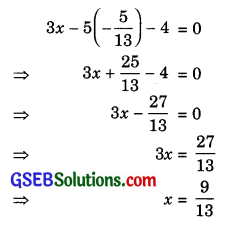So, the solution of the given system of equations isII. By Substitution method:
The given system of equation is
3x – 5y – 4 = 0  …….(1)
9x = 2y + 7  …….(2)
From equation (2),
x = $$\frac{2 y+7}{9}$$ …….(3)
Substituting this value of a; in equation (1), we get
3$$\frac{2 y+7}{9}$$ – 5y – 4 = 0
⇒ $$\frac{2 y+7}{3}$$ 5y – 4 = 0
⇒ 2y + 7 – 15y – 12 = 0
⇒ -13y – 5 = 0
⇒ 13y = – 5
⇒ y = $$\frac{-5}{13}$$
Substituting this value ofy in equation (3), we get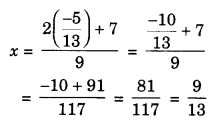So, the solution of the given system of equations is x= $$\frac{9}{13}$$ , y = $$\frac{-5}{13}$$
Verification:
Substituting x = $$\frac{9}{13}$$, y = $$\frac{-5}{13}$$, we find that both the equation (1) and (2) are satisfied as shown below: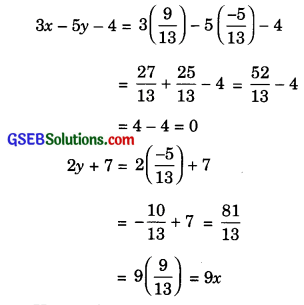Hence, the solution is correct.4. $$\frac{x}{2}+\frac{2 y}{3}$$ = -1 and x – $$\frac{y}{3}$$ – 3
I. By Elimination method:
The given system of equation is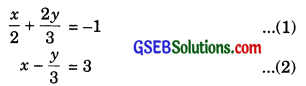Multiplying equation (2) by 2, we get
2x – $$\frac{2y}{3}$$ = 6 …..(3)
Adding equation (1) and equation (3), we getSubstituting this value of x in equation (2), we get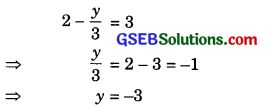So, the solution of the given system of equations is x = 2, y = -3.II. By substitution method:
The given system of equation is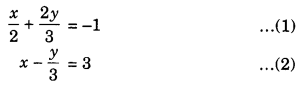From equation (2),
x = $$\frac{y}{3}$$ + 3 …..(3)
Substitute this value of x in equation (1), we get 2 ySubstituting this value ofy in equation (3), we get
x = –$$\frac{3}{3}$$ + 3 = -1 + 3 = 2
So, the solution of the given system of equations is
x = 2, y = -3
Verification:
Substituting x = 2, y = -3, we find that both the equations (1) and (2) are satisfied as shown below: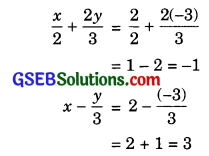Hence, the solution is correct.
The substitution method was most efficient in this case.Question 2.
Form the pair of linear equations in the following problems, and find their solutions (if they exist) by the elimination method:

1. If we add 1 to the numerator and subtract 1 from the denominator, a fraction reduces to 1. It becomes $$\frac{1}{2}$$ if we only add 1 to the denominator. What is the fraction?

2. Five years ago, Nuri was thrice as old as Sonu. Ten years later. Nuri will be twice as old as Sonu. How old are Nuri and Sonu?

3. The sum of the digits of a two-digit number is 9. Also, nine times this number is twice the number obtained by reversing the order of the number. Find the number.

4. Meena went to a bank to withdraw ₹ 2000. She asked the cashier to give her ₹ 50 and ₹ 100 notes only. Meena got 25 notes in all. Find how many notes of ₹ 50 and ₹ 100 she received.

5. A lending library has a fixed charge for the first three days and an additional charge for each day thereafter. Saritha paid ₹ 27 for a book kept for seven days, while Susy paid ₹ 21 for the book she kept five days. Find the fixed charge and the charge for each extra day.

Solution:
1. Let the fraction be $$\frac{x}{y}$$.
Then, according to the question,⇒ x + 1 = y – 1
2x = y + 1
⇒ x – y = -2 …..(1)
⇒ 2x – y = 1 …..(2)
Subtracting equation (1) from equation (2), we get
x = 3
Substituting this value of x in equation (1), we get
3 – y = -2
⇒ y = 3 + 2
⇒ y = 5
Hence, the required fraction is $$\frac{3}{5}$$.Verification:
Substituting the values of x = 3 and y = 5, we find that both the equations cases are satisfied as shown below: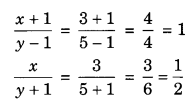Hence, the solution is correct.

2. Let Nuri and Sonu be x years and y years old respectively at present.
Then, according to the question,
x – 5 = 3(y – 5)
x + 10 = 2(y + 10)
⇒ x – 5 = 3y – 15
x + 10 = 2y + 20
⇒ x – 3y = -10 …(1)
x – 2y = 10 …(2)
Subtracting equation (2) from equation (1), we get
-y = -20
⇒ y = 20
Substituting this value ofy in equation (2), we get
x – 2(20) = 10
⇒ x – 40 = 10
⇒ x = 40 + 10
⇒ x = 50
Hence, Nuri and Sonu are 50 years and 20 years old respectively at present.Verification:
Substituting the values of x = 50 and y = 20, we find that both the equations (1) and (2) are satisfied as shown below:
x – 3y = 50 – 3(20)
= 50 – 60 = -10
x – 2y = 50 – 2(20)
= 50 – 40 = 10
Hence, the solution is correct.

3. Let the unit’s digit and the ten’s digit in the two-digit number be x and y respectively.
Then, the number = 10y + x
Also, the number obtained by reversing the
order of the digits = 10x + y
According to the question,
x + y = 9 …(1)
9(10y + x) = 2(10x + y)
⇒ 90y + 9x = 20x + 2y
⇒ 11x – 88y = 0
⇒ x – 8y = 0 …(2)
Subtracting equation (2), from equation (1), we get
9y = 9
⇒ y = $$\frac{9}{9}$$ = 1
Substituting this value of y in equation (1), we get
x + 1 = 9
⇒ x = 9 – 1 = 8
Hence, the required number is 18.
Verification:
Substituting x = 8 and y = 1, we find that both the equations (1) and (2) are satisfied as shown below:
x + y = 8 + 1 = 9
x – 8y = 8 – 8(1) = 0
Hence, the solution is correct.4. Suppose that Meena received x notes of ₹ 50 and y notes of ₹ 100.
Then, according to the question,
x + y = 25 …(1)
50x + 100y = 2000
⇒ x + 2y = 40 …(2)
Subtracting equation (1) from equation (2), we get
y = 15
Substituting this value of y in equation (1), we get
x + 15 = 25
⇒ x = 25 – 15 = 10
Hence, Meena received 10 notes of ₹ 50 and 15 notes of ₹ 100.
Verification:
Substituting x = 10 and y = 15, we find that both the equations (1) and (2) are satisfied as shown below:
x + y = 10 + 15 = 25
x + 2y = 10 + 2(15)
= 10 + 30 = 40
Hence, the solution is correct.5. Let the fixed charge be ₹ a and the charge for each extra day be ₹ b.
Then, according to the question,
a + 46 = 27 …(1)
(∵ Extra days = 7 – 3 = 4)
a + 2b = 21 …(2)
(∵ Extra days = 5 – 3 = 2)
Subtracting equation (2) from equation (1), we get
2b = 6
⇒ b = $$\frac{6}{2}$$ = 3
Substituting this value of 6 in equation (2), we get
a + 2(3) = 21
⇒ a + 6 = 21
⇒ a = 21 – 6 = 15
Hence, the fixed charge is ₹ 15 and the charge for each extra day is ₹ 3.Verification:
Substituting a = 15 and 6 = 3, we find that both the equations (1) and (2) are satisfied as shown below:
a + 46 = 15 + 4(3)
= 15 + 12 = 27
a + 26 = 15 + 2(3)
= 15 + 6 = 21
Hence, the solution is correct.